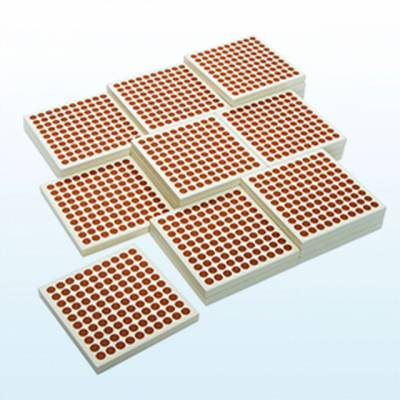CH - Mathematics
45 Wooden Hundred Squares
R 573CH - Mathematics
R 782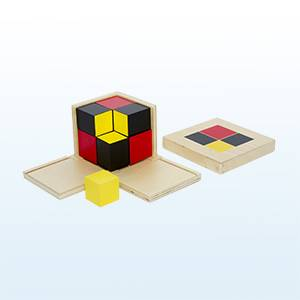CH - Mathematics
Algebraic Binomial Cube
R 782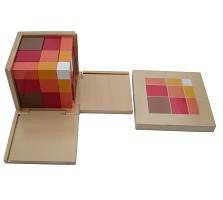CH - Mathematics
Arithmetic Trinomial Cube
R 869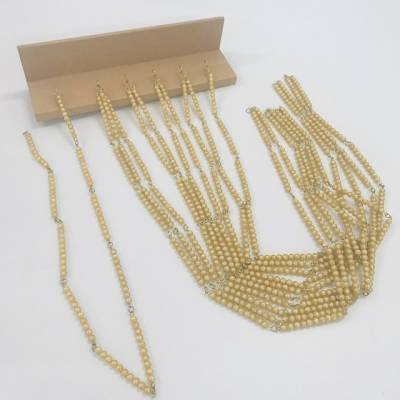CH - Mathematics
R 590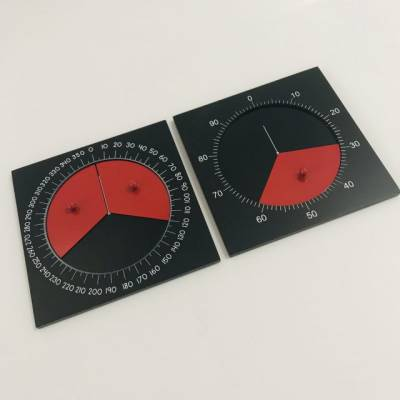CH - Mathematics
Centisimal Frame & Instrument for Measuring Angles
R 173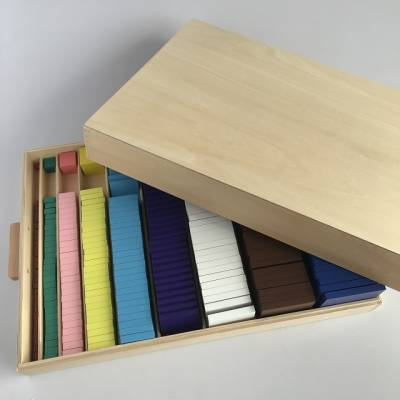CH - Mathematics
Cubing and Cube Root Material
R 3,825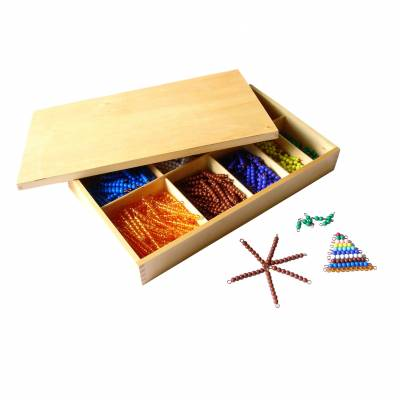CH - Mathematics